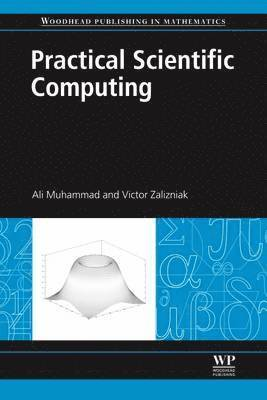Data & IT

# Practical Scientific Computing

Pocket

1049:-

Funktionen begränsas av dina webbläsarinställningar (t.ex. privat läge).

Fri frakt för medlemmar vid köp för minst 199:-

• 208 sidor
• 2011
Scientific computing is about developing mathematical models, numerical methods and computer implementations to study and solve real problems in science, engineering, business and even social sciences. Mathematical modelling requires deep understanding of classical numerical methods. This essential guide provides the reader with sufficient foundations in these areas to venture into more advanced texts.

The first section of the book presents numEclipse, an open source tool for numerical computing based on the notion of MATLAB. numEclipse is implemented as a plug-in for Eclipse, a leading integrated development environment for Java programming. The second section studies the classical methods of numerical analysis. Numerical algorithms and their implementations are presented using numEclipse.

Practical scientific computing is an invaluable reference for undergraduate engineering, science and mathematics students taking numerical methods courses. It will also be a useful handbook for postgraduate researchers and professionals whose work involves scientific computing.

• An invaluable reference for undergraduate engineering, science and mathematics students taking numerical methods courses
• Guides the reader through developing a deep understanding of classical numerical methods
• Features a comprehensive analysis of numEclipse including numerical algorithms and their implementations